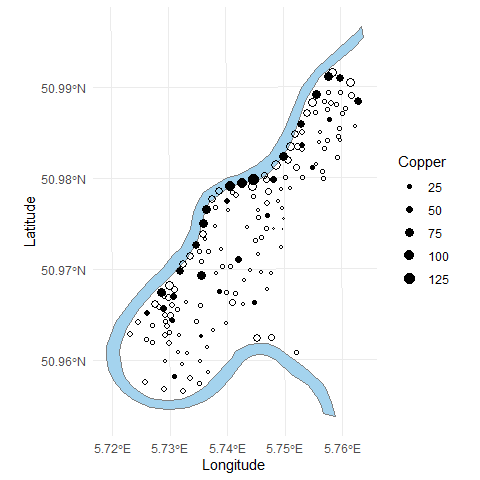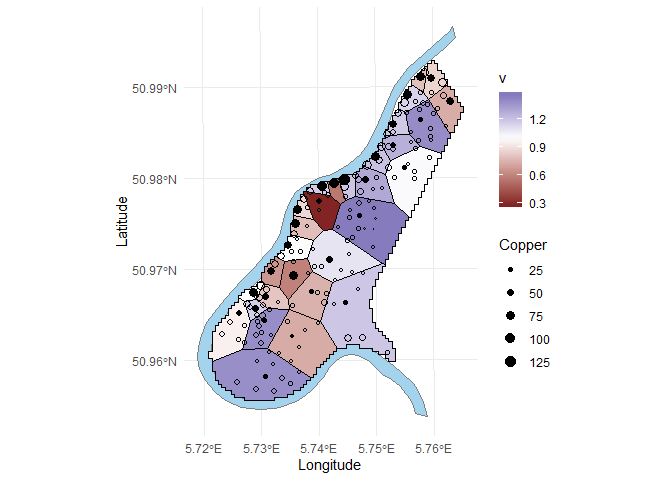Introduction

Geographical data are generally auto-correlated. This is why it is preferable to avoid the measurement of neighboring units. We propose a new method for selecting spread samples from a finite spatial population with equal or unequal inclusion probabilities. The proposed method is called wave and stands for Weakly Associated Vectors. It is based on the definition of the contiguity structure by using a very dense stratification. The method satisfies exactly the inclusion probabilities and provides samples that are very well spread. This document propose an introduction to understand how to use the function wave().

Data generation

We use the data set meuse from the package sp, describe by the following statement. This data set gives locations and topsoil heavy metal concentrations, along with a number of soil and landscape variables at the observation locations, collected in a flood plain of the river Meuse, near the village of Stein (NL). As it is explained by Grafström and Tillé (2013), we proposed to generate the inclusion probabilities proportional to the copper concentration, a variable that have a strong spatial correlation.

# install.packages(sp)
library(sp)
library(sf)
#> Linking to GEOS 3.6.1, GDAL 2.2.3, PROJ 4.9.3
library(sampling)
data("meuse")
data("meuse.riv")
meuse.riv <- meuse.riv[which(meuse.riv[,2] < 334200 & meuse.riv[,2] > 329400),]
meuse_sf <- st_as_sf(meuse, coords = c("x", "y"), crs = 28992, agr = "constant")

X <- scale(as.matrix(meuse[,1:2]))
pik <- inclusionprobabilities(meuse$copper,30) Sample selection The sample selection is easily performed by the function wave(). s <- wave(X,pik) sum(s) #>  30 library(ggplot2) p <- ggplot()+ geom_sf(data = meuse_sf,aes(size=copper),show.legend = 'point',shape = 1,stroke = 0.3)+ geom_polygon(data = data.frame(x = meuse.riv[,1],y = meuse.riv[,2]), aes(x = x,y = y), fill = "lightskyblue2", colour= "grey50")+ geom_point(data = meuse, aes(x = x,y = y,size = copper), shape = 1, stroke = 0.3)+ geom_point(data = meuse[which(s == 1),], aes(x = x,y = y,size = copper), shape = 16)+ labs(x = "Longitude", y = "Latitude", title = NULL, size = "Copper", caption = NULL)+ scale_size(range = c(0.5, 3.5))+ theme_minimal() pSpatial balance Voronoï polygons One way of measuring the spread of a sample was developed by Stevens and Olsen (2004) and then suggested by Grafström, Lundström, and Schelin (2012). It is based on the Voronoï polygons and is given by $B(\bf s) = \frac{1}{n}\sum_{i \in s} (v_i - 1)^2$ where $$v_i$$ is equal to the sum of the inclusion probabilities inside the $$i$$th polygons and $$\bf s$$ is the vector of size $$N$$ with elements equal 0 or 1. This quantity is implemented in the package BalancedSampling with the function sb(). We calculate the values of the $$v_k$$ with the function sb_vk. The closer $$B(\bf s)$$ is to zero, the better is the spatial balance of the sample. Graphically, we obtain the following plot. library(sp) library(sampling) library(ggvoronoi) data("meuse") data("meuse.area") v <- sb_vk(pik,as.matrix(meuse[,1:2]),s) meuse$v <- v

p <- p + geom_voronoi(data = meuse[which(s == 1),],
aes(x = x,y = y,fill = v),
outline =as.data.frame(meuse.area),
size = 0.1,
colour = "black")+
geom_point(data = meuse,
aes(x = x,y = y,size = copper),
shape = 1,
stroke = 0.3)+
geom_point(data = meuse[which(s == 1),],
aes(x = x,y = y,size = copper),
shape = 16)+
pBalancedSampling::sb(pik,as.matrix(meuse[,1:2]),which(s == 1))
#>  0.08031111

Moran index

Another way to estimate the spatial spread is developed by Tillé et al. (2018), it uses a corrected version of the traditional Moran’s $$I$$ index. This estimator use spatial weights $$w_{ij}$$ that indicates how a unit $$i$$ is close from the unit $$j$$. Such matrix is supposed to include inclusion probabilities in its computation, hence, the spatial weights matrix $$\bf W$$ is generally not symmetric. The spatial balance measure is given by

$I_B =\frac{(\bf s-\bf \bar{s}_w)^\top \bf W (\bf s-\bf \bar{s}_w)}{\sqrt{(\bf s-\bf \bar{s}_w)^\top \bf D (\bf s-\bf \bar{s}_w) (\bf s-\bf \bar{s}_w)^\top \bf B (\bf s-\bf \bar{s}_w) }},$ where $$\bf D$$ is the diagonal matrix containing the $$w_i$$, $\bf \bar{s}_w = \bf 1 \frac{\bf s^\top \bf W \bf 1}{\bf 1^\top \bf W \bf 1},$ and $\bf B = \bf W^\top \bf D^{-1} \bf W - \frac{\bf W^\top \bf 1\bf 1^\top \bf W}{\bf1^\top \bf W \bf 1}.$

The Moran’s $$I$$ index is implemented in the function IB(). It is possible to specify your own spatial weights with the argument W. There is no natural way of defining $$\bf W$$, here we propose to consider for each unit only the neighbour such that the sum of the inclusion probabilities of the stratum sum up to 1. It is implemented in the function wpik(). Another way of estimating the spatial weights is developed by Tillé et al. (2018) and use the inverse of the inclusion probabilities $$1/\pi_i$$ to estimate the neighbours of the unit $$i$$. It is implemented in the function wpikInv(). As explain by Tillé et al. (2018) $$w_{ii}$$ is supposed to be equal to 0 for all $$i \in U$$. By construction the function wpik does not return the diagonal equal to zero. So if we want to calculate the Moran’s I index with wpik, we need to subtract the diagonal of the returned matrix.

W <- wpik(X,pik)
W <- W - diag(diag(W))
IB(W,s)
#>  -0.3786126

W1 <- wpikInv(X,pik)
IB(W1,s)
#>  -0.3872553